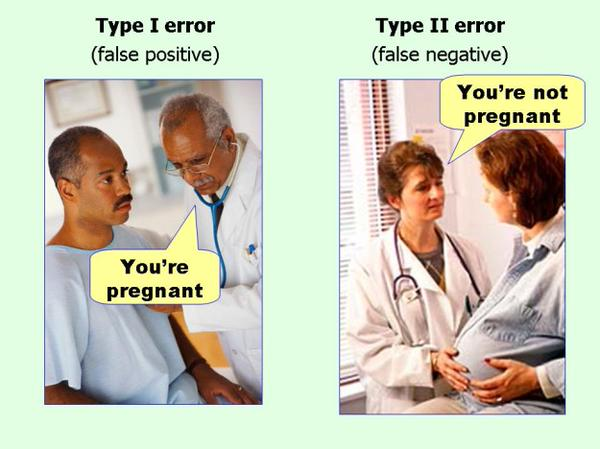# Python/nltk: Naive vs Naive Bayes vs Decision Tree

Last week I wrote a blog post describing a decision tree I’d trained to detect the speakers in a How I met your mother transcript and after writing the post I wondered whether a simple classifier would do the job.

The simple classifier will work on the assumption that any word followed by a ":" is a speaker and anything else isn’t. Here’s the definition of a NaiveClassifier:

``````import nltk
from nltk import ClassifierI

class NaiveClassifier(ClassifierI):
def classify(self, featureset):
if featureset['next-word'] == ":":
return True
else:
return False``````

As you can see it only implements the classify method and executes a static check.

While reading about ways to evaluate the effectiveness of text classifiers I came across Jacob Perkins blog which suggests that we should measure two things: precision and recall.

• Higher precision means less false positives, while lower precision means more false positives.

• Higher recall means less false negatives, while lower recall means more false negatives.

If (like me) you often get confused between false positives and negatives the following photo should help fix that:

</p>I wrote the following function (adapted from Jacob’s blog post) to calculate precision and recall values for a given classifier:

``````import nltk
import collections

def assess_classifier(classifier, test_data, text):
refsets = collections.defaultdict(set)
testsets = collections.defaultdict(set)
for i, (feats, label) in enumerate(test_data):
observed = classifier.classify(feats)

speaker_precision = nltk.metrics.precision(refsets[True], testsets[True])
speaker_recall = nltk.metrics.recall(refsets[True], testsets[True])

non_speaker_precision = nltk.metrics.precision(refsets[False], testsets[False])
non_speaker_recall = nltk.metrics.recall(refsets[False], testsets[False])

return [text, speaker_precision, speaker_recall, non_speaker_precision, non_speaker_recall]``````

Now let’s call that function with each of our classifiers:

``````import json

from sklearn.cross_validation import train_test_split
from himymutil.ml import pos_features
from himymutil.naive import NaiveClassifier
from tabulate import tabulate

with open("data/import/trained_sentences.json", "r") as json_file:

tagged_sents = []
for sentence in json_data:
tagged_sents.append([(word["word"], word["speaker"]) for word in sentence["words"]])

featuresets = []
for tagged_sent in tagged_sents:
untagged_sent = nltk.tag.untag(tagged_sent)
for i, (word, tag) in enumerate(tagged_sent):
featuresets.append( (pos_features(untagged_sent, i), tag) )

train_data,test_data = train_test_split(featuresets, test_size=0.20, train_size=0.80)

table = []
table.append(assess_classifier(NaiveClassifier(), test_data, "Naive"))
table.append(assess_classifier(nltk.NaiveBayesClassifier.train(train_data), test_data, "Naive Bayes"))
table.append(assess_classifier(nltk.DecisionTreeClassifier.train(train_data), test_data, "Decision Tree"))

print(tabulate(table, headers=["Classifier","speaker precision", "speaker recall", "non-speaker precision", "non-speaker recall"]))``````

I’m using the tabulate library to print out a table showing each of the classifiers and their associated value for precision and recall. If we execute this file we’ll see the following output:

``````\$ python scripts/detect_speaker.py
Classifier       speaker precision    speaker recall    non-speaker precision    non-speaker recall
-------------  -------------------  ----------------  -----------------------  --------------------
Naive                     0.9625            0.846154                 0.994453              0.998806
Naive Bayes               0.674603          0.934066                 0.997579              0.983685
Decision Tree             0.965517          0.923077                 0.997219              0.998806``````

The naive classifier is good on most measures but makes some mistakes on speaker recall - we have 16% false negatives i.e. 16% of words that should be classified as speaker aren’t.

Naive Bayes does poorly in terms of speaker false positives - 1/3 of the time when we say a word is a speaker it actually isn’t.

The decision tree performs best but has 8% speaker false negatives - 8% of words that should be classified as speakers aren’t.

The code is on github if you want to play around with it.